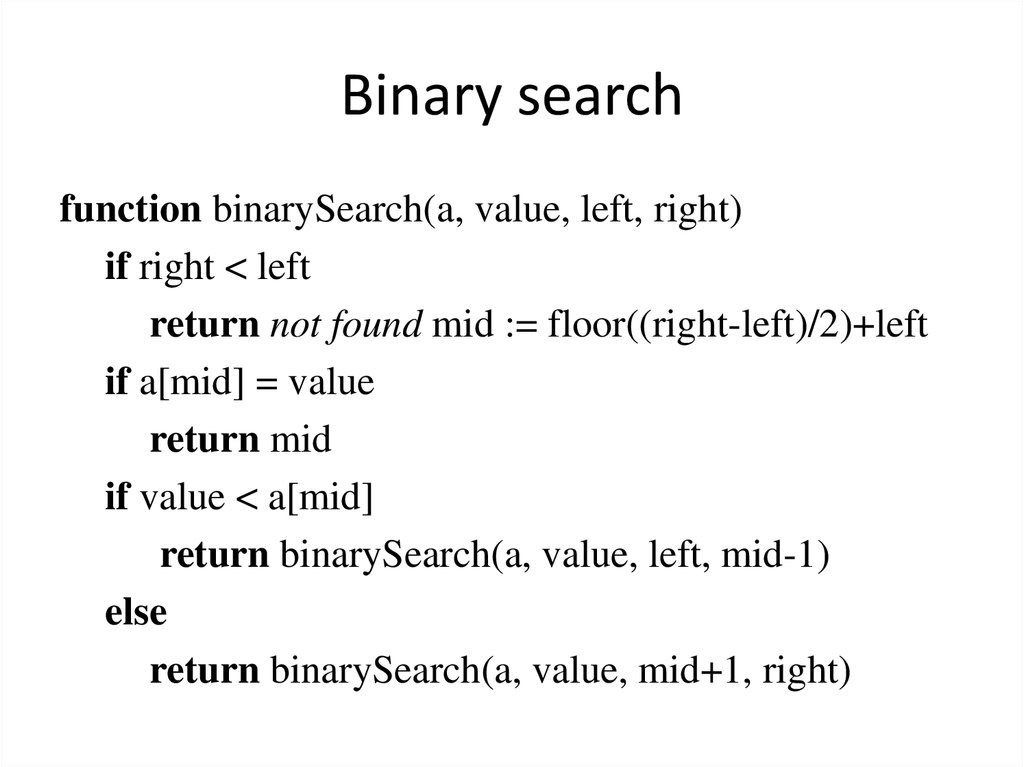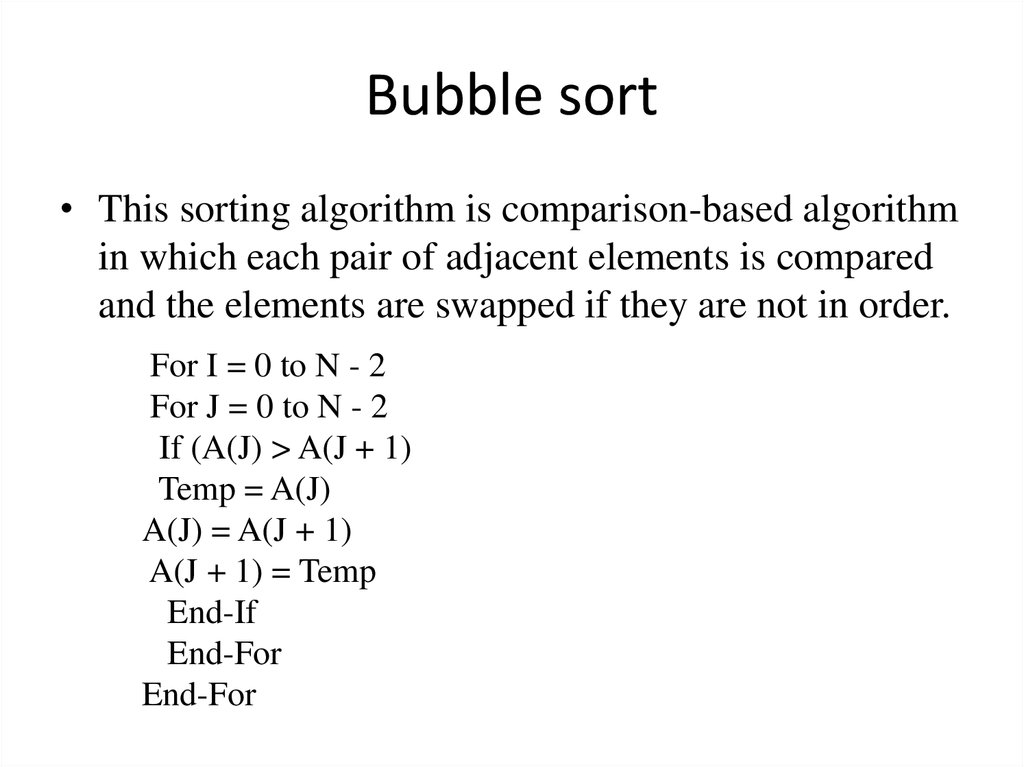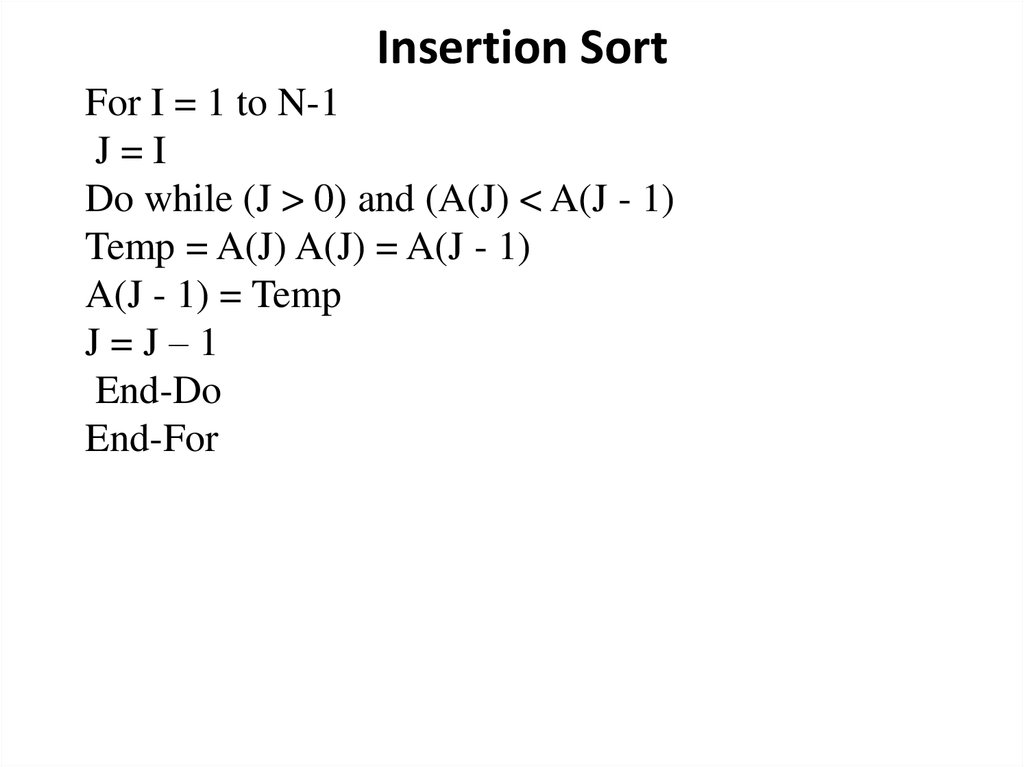# Binary search

## 1. Binary search

function binarySearch(a, value, left, right)
if right < left
if a[mid] = value
return mid
if value < a[mid]
return binarySearch(a, value, left, mid-1)
else
return binarySearch(a, value, mid+1, right)

## 2. Bubble sort

• This sorting algorithm is comparison-based algorithm
in which each pair of adjacent elements is compared
and the elements are swapped if they are not in order.
For I = 0 to N - 2
For J = 0 to N - 2
If (A(J) > A(J + 1)
Temp = A(J)
A(J) = A(J + 1)
A(J + 1) = Temp
End-If
End-For
End-For

## 3.

Insertion Sort
For I = 1 to N-1
J=I
Do while (J > 0) and (A(J) < A(J - 1)
Temp = A(J) A(J) = A(J - 1)
A(J - 1) = Temp
J=J–1
End-Do
End-For